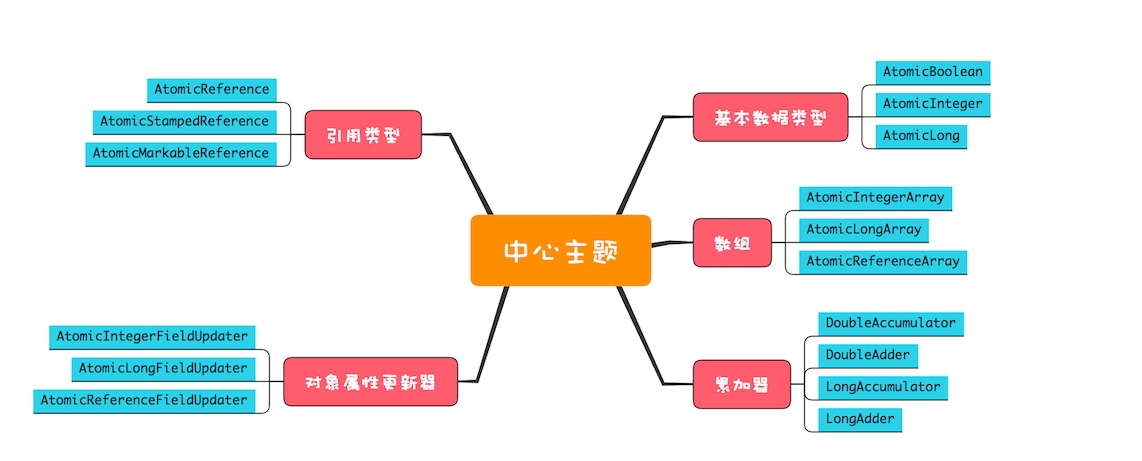## （十九）CAS和Atomic

2021/1/28 23:38:54 文章标签:

``````public class Test {
long count = 0;
void add10K() {
int idx = 0;
while(idx++ < 10000) {
count += 1;
}
}
}
``````

``````public class Test {
AtomicLong count =
new AtomicLong(0);
void add10K() {
int idx = 0;
while(idx++ < 10000) {
count.getAndIncrement();
}
}
}
``````

## 无锁方案的实现原理

``````class SimulatedCAS{
int count；
synchronized int cas(
int expect, int newValue){
// 读目前count的值
int curValue = count;
// 比较目前count值是否==期望值
if(curValue == expect){
// 如果是，则更新count的值
count = newValue;
}
// 返回写入前的值
return curValue;
}
}
``````

``````class SimulatedCAS{
volatile int count;
// 实现count+=1
addOne(){
do {
newValue = count+1; //①
}while(count !=
cas(count,newValue) //②
}
// 模拟实现CAS，仅用来帮助理解
synchronized int cas(
int expect, int newValue){
// 读目前count的值
int curValue = count;
// 比较目前count值是否==期望值
if(curValue == expect){
// 如果是，则更新count的值
count= newValue;
}
// 返回写入前的值
return curValue;
}
}
``````

## 看Java如何实现原子化的count += 1

``````final long getAndIncrement() {
return unsafe.getAndAddLong(
this, valueOffset, 1L);
}
``````

unsafe.getAndAddLong()方法的源码如下，该方法首先会在内存中读取共享变量的值，之后循环调用compareAndSwapLong()方法来尝试设置共享变量的值，直到成功为止。compareAndSwapLong()是一个native方法，只有当内存中共享变量的值等于expected时，才会将共享变量的值更新为x，并且返回true；否则返回fasle。compareAndSwapLong的语义和CAS指令的语义的差别仅仅是返回值不同而已。

``````public final long getAndAddLong(
Object o, long offset, long delta){
long v;
do {
// 读取内存中的值
v = getLongVolatile(o, offset);
} while (!compareAndSwapLong(
o, offset, v, v + delta));
return v;
}
//原子性地将变量更新为x
//条件是内存中的值等于expected
//更新成功则返回true
native boolean compareAndSwapLong(
Object o, long offset,
long expected,
long x);
``````

``````do {
// 获取当前值
oldV = xxxx；
// 根据当前值计算新值
newV = ...oldV...
}while(!compareAndSet(oldV,newV);
``````

## 原子类概览

Java SDK并发包里提供的原子类内容很丰富，我们可以将它们分为五个类别：原子化的基本数据类型、原子化的对象引用类型、原子化数组、原子化对象属性更新器原子化的累加器。这五个类别提供的方法基本上是相似的，并且每个类别都有若干原子类，你可以通过下面的原子类组成概览图来获得一个全局的印象。下面我们详细解读这五个类别。### 1. 原子化的基本数据类型

``````getAndIncrement() //原子化i++
getAndDecrement() //原子化的i--
incrementAndGet() //原子化的++i
decrementAndGet() //原子化的--i
//当前值+=delta，返回+=前的值
getAndAdd(delta)
//当前值+=delta，返回+=后的值
addAndGet(delta)
//CAS操作，返回是否成功
compareAndSet(expect, update)
//以下四个方法
//新值可以通过传入func函数来计算
getAndUpdate(func)
updateAndGet(func)
getAndAccumulate(x,func)
accumulateAndGet(x,func)
``````

### 2. 原子化的对象引用类型

``````boolean compareAndSet(
V expectedReference,
V newReference,
int expectedStamp,
int newStamp)
``````

AtomicMarkableReference的实现机制则更简单，将版本号简化成了一个Boolean值，方法签名如下：

``````boolean compareAndSet(
V expectedReference,
V newReference,
boolean expectedMark,
boolean newMark)
``````

### 4. 原子化对象属性更新器

``````public static
AtomicXXXFieldUpdater
newUpdater(Class tclass,
String fieldName)
``````

``````boolean compareAndSet(
T obj,
int expect,
int update)
``````

### 5. 原子化的累加器

DoubleAccumulator、DoubleAdder、LongAccumulator和LongAdder，这四个类仅仅用来执行累加操作，相比原子化的基本数据类型，速度更快，但是不支持compareAndSet()方法。如果你仅仅需要累加操作，使用原子化的累加器性能会更好。

## 总结

Java提供的原子类能够解决一些简单的原子性问题，但你可能会发现，上面我们所有原子类的方法都是针对一个共享变量的，如果你需要解决多个变量的原子性问题，建议还是使用互斥锁方案。原子类虽好，但使用要慎之又慎。

## 课后思考

``````public class SafeWM {
class WMRange{
final int upper;
final int lower;
WMRange(int upper,int lower){
//省略构造函数实现
}
}
final AtomicReference
rf = new AtomicReference<>(
new WMRange(0,0)
);
// 设置库存上限
void setUpper(int v){
WMRange nr;
WMRange or = rf.get();
do{
// 检查参数合法性
if(v < or.lower){
throw new IllegalArgumentException();
}
nr = new
WMRange(v, or.lower);
}while(!rf.compareAndSet(or, nr));
}
}
``````

暂无相关的数据...